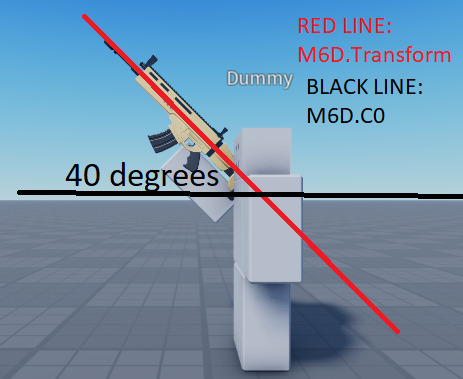# "Canceling" an axis of an animation

What I’m trying to do is to have an animation’s axis being “canceled”, by this I mean:

Original animation:

After “canceling” the X rotation:

I have planned on make use of Motor6D.Transform to get the animation’s rotation, then use Motor6D.C0 to rotate it inversely such that the animation rotation will be overrided by the C0.After overriding with C0:

It works fine when the weapon stays on it’s original position, where `Motor6D.Transform` position = 0,0,0 (The Motor6D is welded to torso)

``````local x,y,z = m6d.Transform:ToOrientation()
m6d.C0 = CFrame.new() * CFrame.Angles(-x, -y, -z)
``````

You can see the animation is supposed to lift the weapon up but it keeps it horizontally. The red line represents the Rotated C0.

However, when the `M6D.Transform` is not` 0,0,0`, this happens.

This can be well explained with some math, because how the C0 has been rotated. Notice how the C0 now acts like a slope. So normally when the animation moves back, it now also moves back but along with the slope.

We have to set the location of the C0 instead of just rotation. (Red box)

Since the distance moved is always the same, we can determine that it is an isosceles triangle. The base of this isosceles triangle is the distance that we need to move.

``````local x,y,z = m6d.Transform:ToOrientation()
local pos = m6d.Transform.Position
m6d.C0 = CFrame.new(0 , 2 * pos.z * math.cos( (180-x) /2) , 0) * CFrame.Angles(-x, -y, -z)
``````

However the result seems to be still off and the offset remains, am I getting anything wrong here?